# What is the Oracle Optimizer and How Cost-Based Optimizer Works ? | Oracle Database Performance Tuning Tutorial -3

Hi,

I will explain What is the Oracle Optimizer and How Cost-Based Optimizer Works in this article.

You can read the following article before this.

Performance Tuning Features and Tools in Oracle | Oracle Database Performance Tuning Tutorial -2

Oracle database has optimizer that determines which execution plan is most efficient and best for the SQL statements. Optimizer checks all available access paths and by factoring in information based on statistics for the tables or indexes accessed by the SQL statement.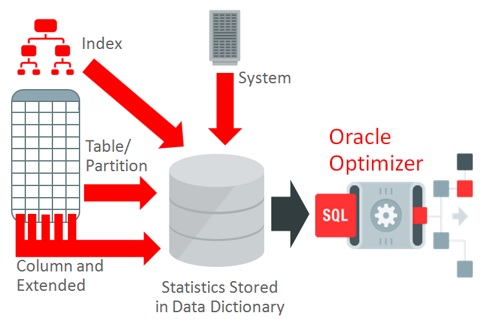Oracle optimizer performs the following steps.

1. It generates lots of plans for the SQL statement based on available access paths.
2.  Oracle optimizer estimates Cost of these potential plans based on Statistics for the data size and storage characteristics of the table and indexes.
3.  Finally it compares the costs of these plans and selects the one execution plan which is the lowest cost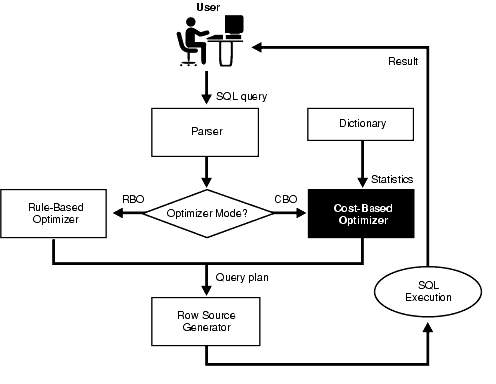Oracle optimizer should find and return the best execution plan every time as quickly as possible for the best performance of SQL Statements.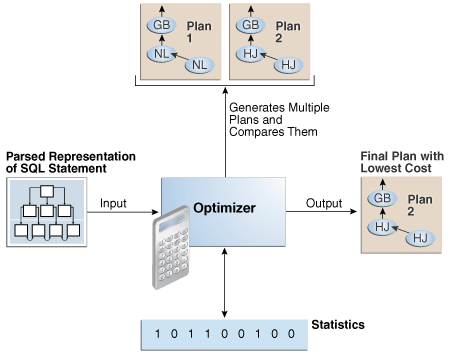For example; You have employee table and execute the following SQL.

`select * from emp where job='MANAGER';`

Oracle optimizer check statistics, Schema information and possible access paths, Oracle found that Only 1% of employees are managers, so Oracle should use the index if exists instead of Full table scan. Optimizer also decides to use this plan like following.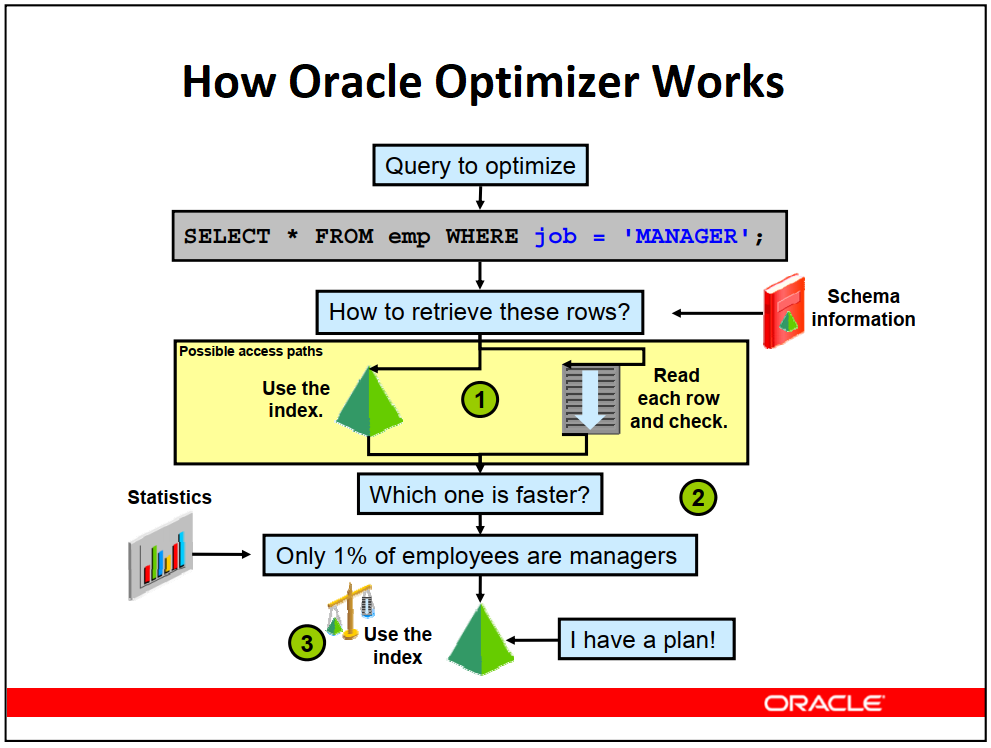But If the 80% of employees were managers, Oracle would use the full table scan instead of Index as follows. Because Using the Full table scan is better than Index scan in this case.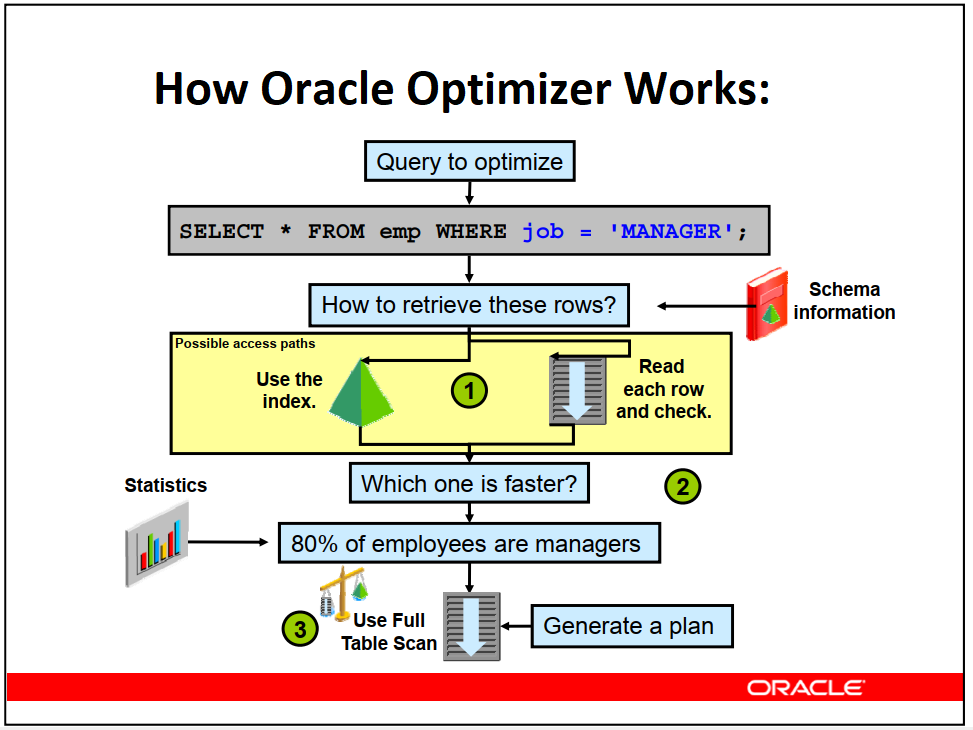There are two types of optimizer in the Oracle database. One type of optimizer is Rule based optimizer (RBO), and the other type of optimizer is Cost based Optimizer (CBO).

Oracle strongly advises the use of Cost based Optimizer, because Rule based optimizer is available for backward compatibility  and will be deprecated in a future release.

### Cost-Based Optimizer

Cost-Based Optimizer consists of the following three main components.

1. Query Transformer
2. Estimator
3. Plan Generator

##### Query Transformer

Firstly Query is transformed a new ( transformed ) query for Estimator usage as follows.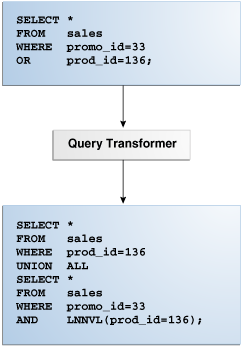##### Estimator

Secondly, Estimator will determine the overall cost of a given execution plan according to some measures like selectivity, cardinality, and cost

These measures are not independent of each other, unlike they are related to each other. The Cost depends on Cardinality and Cardinality is derived from Selectivity.

Estimator: Selectivity

Selectivity is a value between 0.0 and 1.0 that estimated proportion of a row set retrieved by a particular predicate  ( such as last_name = ‘Deveci’ )  or combination of predicates  (such as name = ‘Mehmet Salih ‘ and last_name = ‘Deveci’ ).

High selectivity: Small proportion of rows
Low selectivity: Big proportion of rows

Formula of Selectivity is as follows.Estimator: Cardinality

Cardinality is expected number of rows (  table, view ) retrieved by a particular operation in the execution plan. It is very important during determination of  join, filters, and sort costs.

Formula of Cardinality is as follows.For example; There are 1000 rows in the employees table and number of ‘Mehmet’ value is 50 in the table.

SELECT first_name FROM employees WHERE first_name = ‘Mehmet’;

In this case, Selectivity will be 0.02 , ( Selectivity =1/50=0.02)

and Cardinality will be 20   ( Cardinality = Selectivity * Total number of rows)  ( Cardinality=1000×0.02 = 20 )

Estimator: Cost

The cost is an numeric measure (value) that represents the estimated resource usage for a execution plan. There will be different costs for a specific query according to Environment. There are lots of factors for Oracle optimizer to estimate cost as follows.

• System resources ( I/O, CPU, and memory )
• Access structures
• Estimated number of rows returned (cardinality)
• Size of the initial data sets
• Distribution of the data ( data skew )

1 cost unit = 1 SRds  (  Number of single block reads )

Formula of Cost is as follows.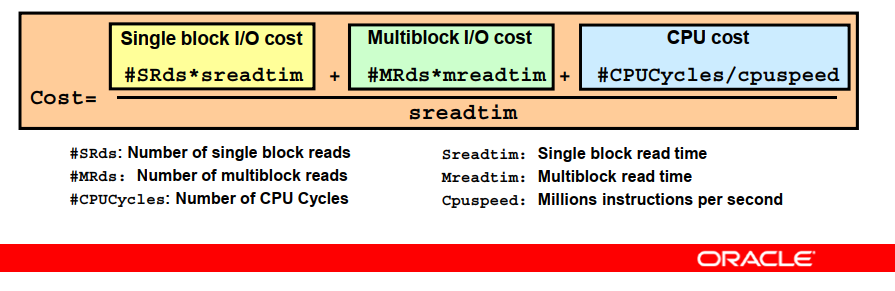##### Plan Generator

Finally, Plan Generator will explore different plans for a query block by trying out different access paths, join methods, and join orders, and then select the one Execution plan with the lowest cost between different possible plans for a given query.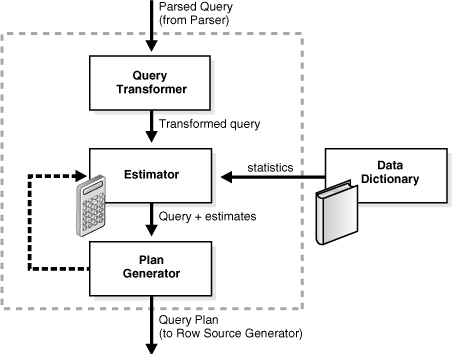There is an internal cutoff of Plan Generator to reduce the number of plans, If the current defined cost is large, then Plan generator will explore more alternative plans.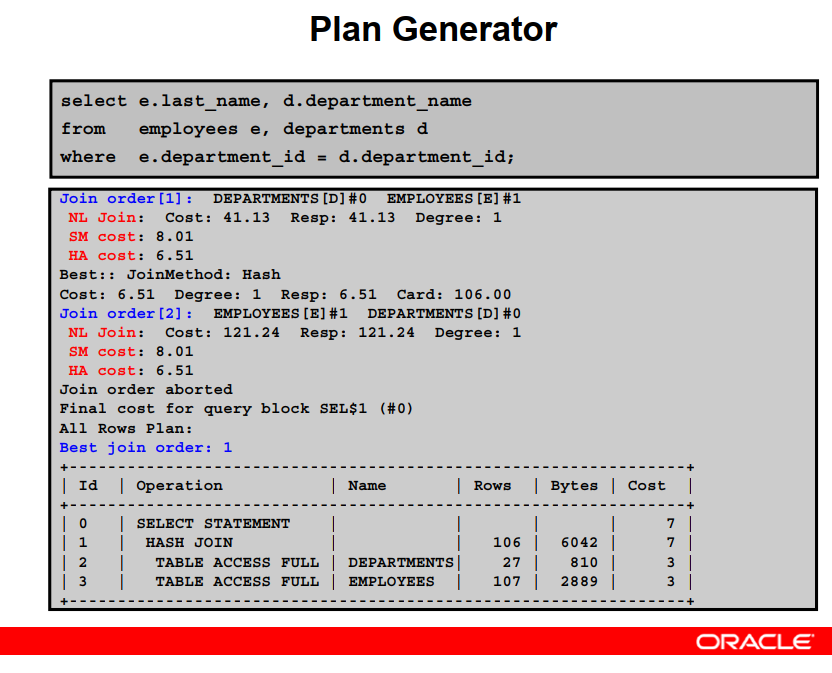There are several parameters for Controlling the Behavior of the Optimizer in Oracle database. There parameters are as follows.

• CURSOR_SHARING
• RESULT_CACHE_MODE
• RESULT_CACHE_MAX_SIZE
• RESULT_CACHE_MAX_RESULT
• RESULT_CACHE_REMOTE_EXPIRATION
• PGA_AGGREGATE_TARGET
• STAR_TRANSFORMATION_ENABLED

I will continue to explain these parameters in the next articles.

Read the following article series to learn about Oracle database Performance Tuning.

https://ittutorial.org/oracle-database-performance-tuning-tutorial-2-performance-tuning-features-and-tools/

#### Do you want to learn Top 30 SQL Tuning Tips and Tricks, then read the following articles.

https://ittutorial.org/oracle-sql-tuning-tips-and-tricks-tutorial-3/

3,599 views last month,  1 views today